# How can Machine Learn? - Nonlinear Transformation

1.首先，非线性模型很显然是线性不可分(non-linear separable)的，如图一所示。可以看到无论怎样都不可能用一条直线分开，图中能刚好用一个圆分割，所以管它叫圈圈可分（Circular Separable），用圆的公式稍做变形可以得到这个圆的公式（1）

hSEP(x)=sign(x21x21+0.6)(1)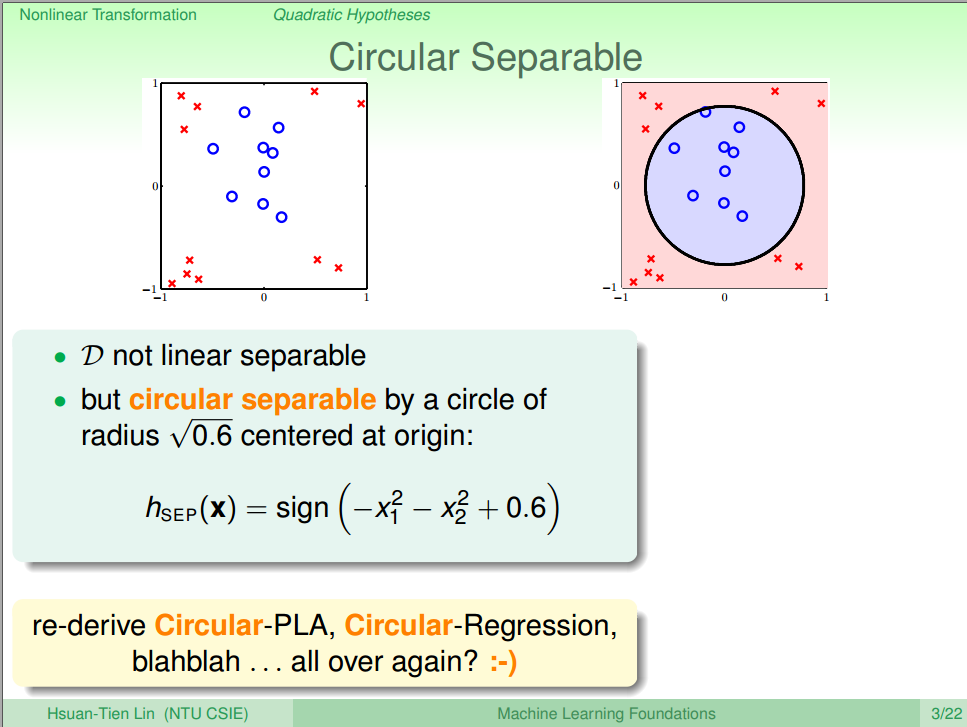2.类似于之前对Linear Model的公式变化，我们对公式（1）稍作调整，可以得到公式类似的结果，如公式（2)所示。 公式（2）的是z 不是 x，这个公式把 {(xn,yn)} $\{(x_n, y_n)\}$ 转换成了线性可分的 {(zn,yn)} $\{(z_n, y_n)\}$ ，这种转换成为特征转化(feature transform)用符号 Φ $\Phi$ 表示，经过特征转换后的图如图二所示。

\begin{align*} h_{SEP}(x) &= sign(-x_1^2 - x_1^2 + 0.6) \ &= sign( \underbrace{0.6}{w_0} \times \underbrace{1}{x_0} + \underbrace{(-1)}{w_1} \times \underbrace{x_1^2}{z_1} + \underbrace{(-1)}{w_2} \times \underbrace{x_2^2}{z_2}) \ &= sign(w^T z) \end{align*} \tag{ 2 $2$}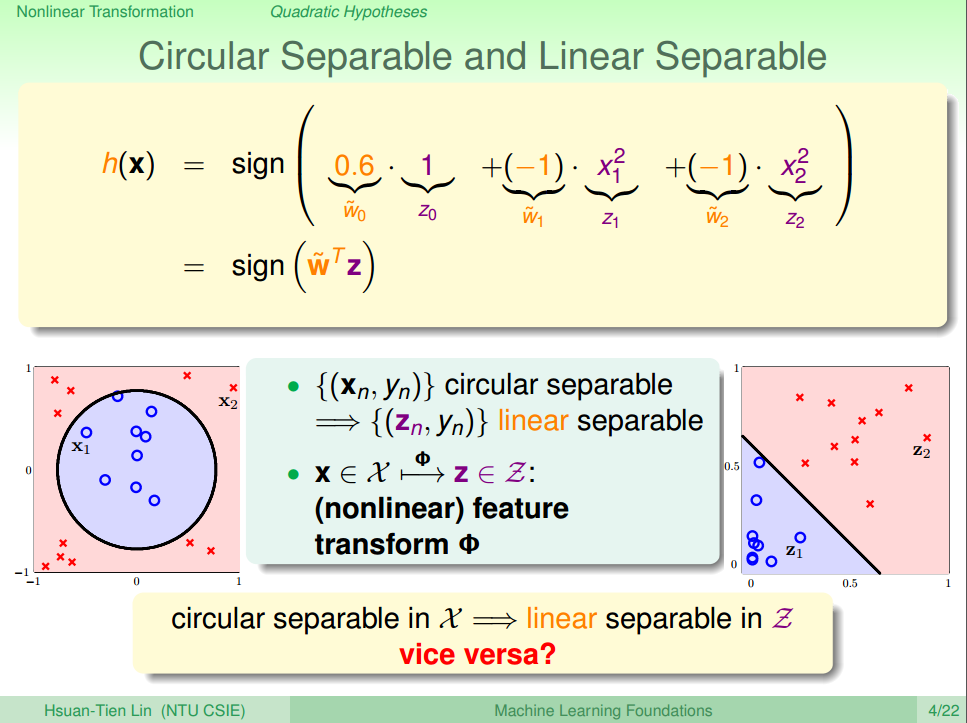3.那么如果能在z空间线性可分，反过来能不能在x空间圆形可分呢？

w 假设函数h(x) 形状
(0.6, -1, -1) sign(0.6-x_1^2-1x_2^2) 圆形（circle）
(0.6, -1, -2) sign(0.6-x_1^2-2x_2^2) 椭圆形（ellipse）
(0.6, -1, +2) sign(0.6-x_1^2+2x_2^2) 抛物线（hyperbola）
(0.6, +1, +2) sign(0.6+x_1^2+2x_2^2) constant:全为+1

Φ2(x)=(1,x1,x2,x21,x22,x1x2,x22)(3)

## 2. Nonlinear Transform

1. 通过特征转换函数 Φ $\Phi$，将在X空间中不可分的数据集 {(xn,yn)} $\{(x_n, y_n)\}$ 转换成在Z空间中可分的数据集 {(zn,yn)} $\{(z_n, y_n)\}$
2. 使用线性分类算法通过数据集 {(zn,yn)} $\{(z_n, y_n)\}$ 获得寻找最优权值向量 w $w$
3. 回到X空间得到需要的返回假设函数 g(x)=sign(wTΦ(xn))$g(x) = sign(w^T \Phi(x_n))$.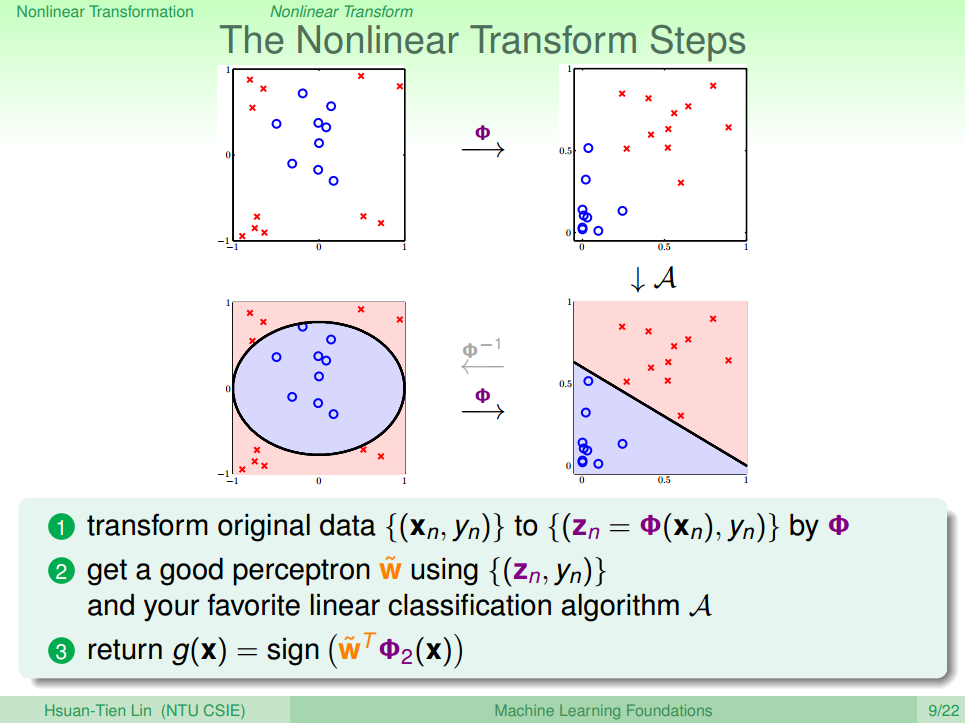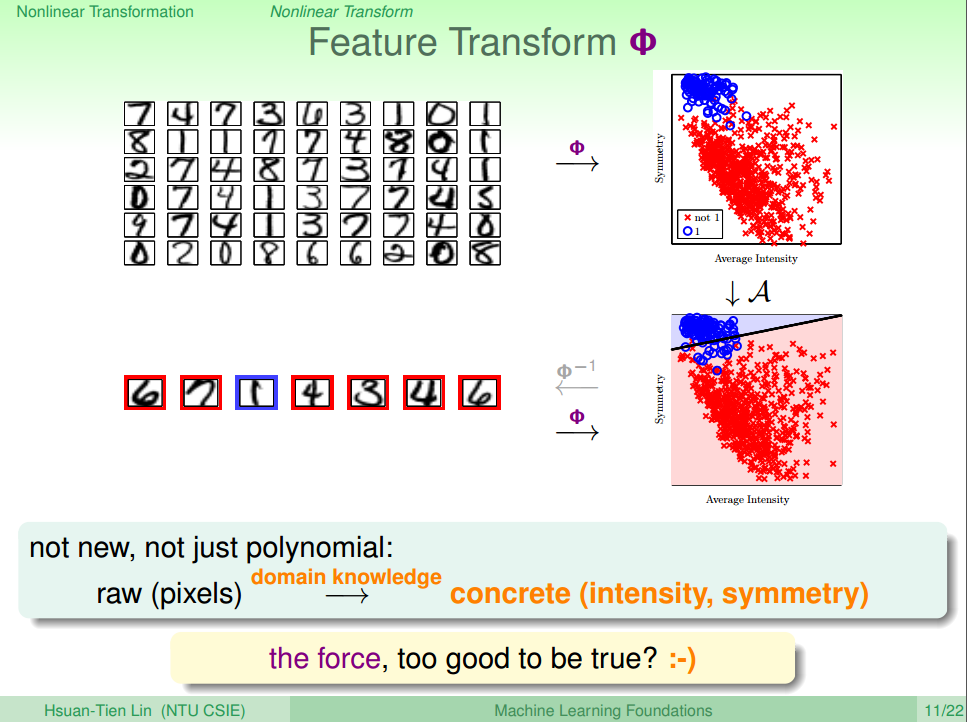## 3. Price of Nonlinear Transform

1.首先，若x空间含有d个类别，即d个特征，特征维度是d维的，那么二次多项式个数，即z空间特征维度是如公式（4）所示

d˘=1+C0d+C1d+d=d+(d+3)2+1=C22+d(4)

d˘=CQQ+d=CdQ+d=O(Qd)(5)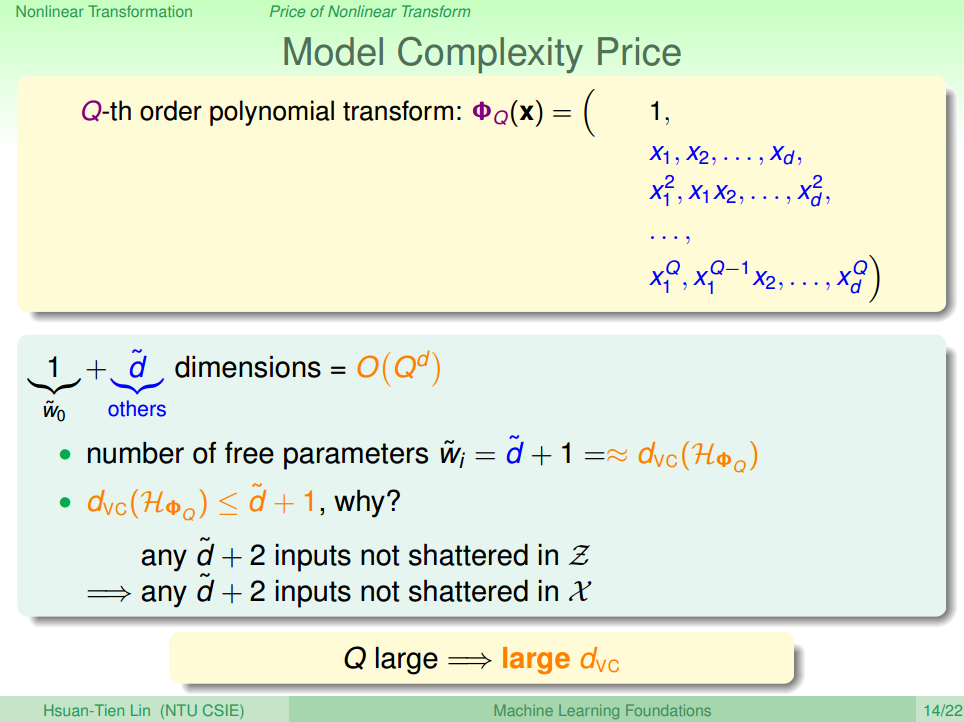2.另一方面，关于泛化能力的问题，因为z域中特征个数随着Q和d增加变得很大，同时权重w也会增大，即自由度增加，VC Dimension增大。根据之前课程的讨论：VC Dimension过大，模型的泛化能力会比较差。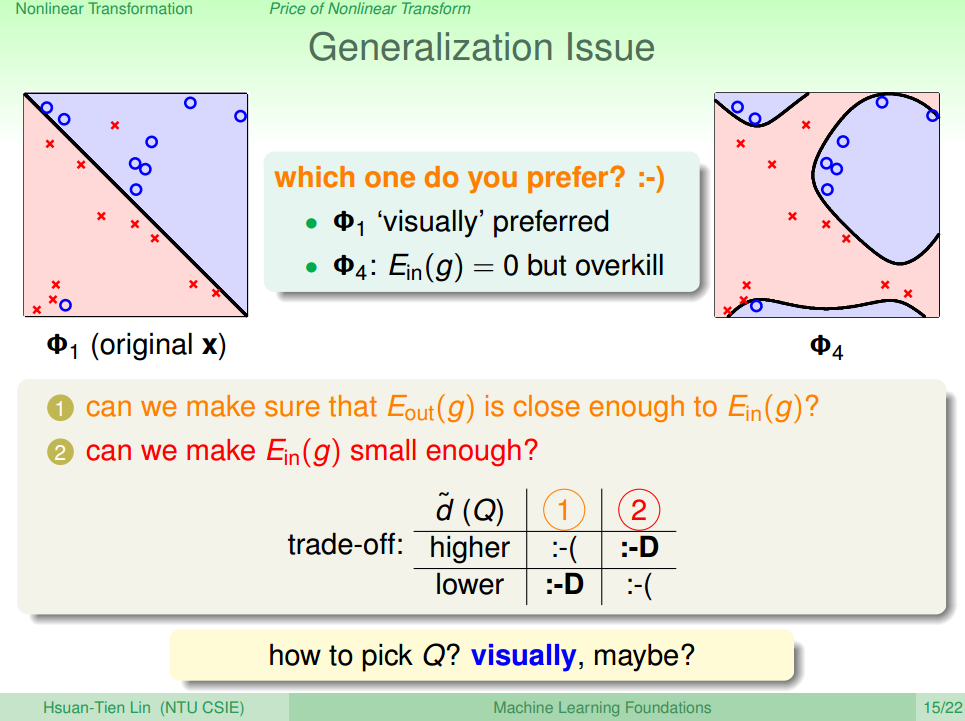1. 从分类结果来看：单从平面上这些训练数据来看，右边的图（四次曲线）的分类效果更好
2. 但是从泛化能力来看的话：四次曲线模型很容易带来过拟合（下一节会讨论）的问题，虽然它的 Ein $E_{in}$ 比较小，从泛化能力上来说，还是左边的分类器更好一些。也就是说VC Dimension过大会带来过拟合问题， d˘+1 $d^˘+1$ 不能太大了。

## 4. Structured Hypothesis Sets

1.首先如果d为1维的话，如公式（6)所示，多项式中只有常数项

Φ0(x)=(1)(6)

2.如果d为2维的时候，如公式（7）所示，多项式中包含了1维的多项式

Φ1(x)=(Φ0(x),x1,x2,,xd)(7)

3.如果d为3维的时候，如公式（8）所示，多项式中包含了2维的多项式

Φ2(x)=(Φ1(x),x21,x1x2,,x2d)(8)

3.以此类推，如果d为Q维的时候，如公式（9）所示，多项式中包含了(Q-1)维的多项式

ΦQ(x)=(ΦQ1(x),xQ1,x1Q1x2,,xQd)(9)

HΦ0HΦ1HΦ2HΦQ(9)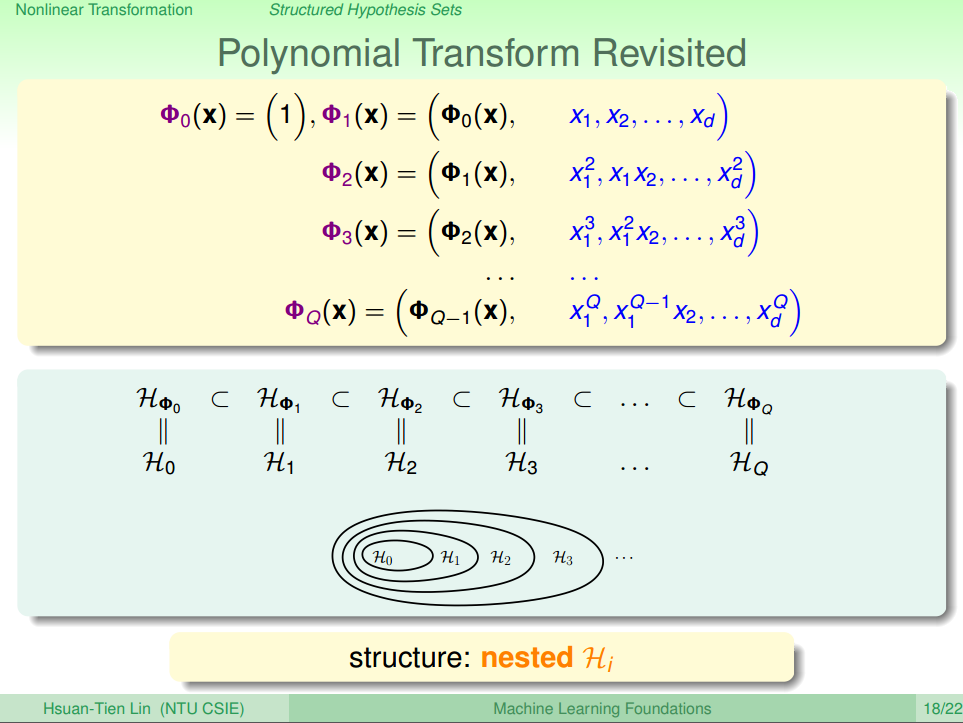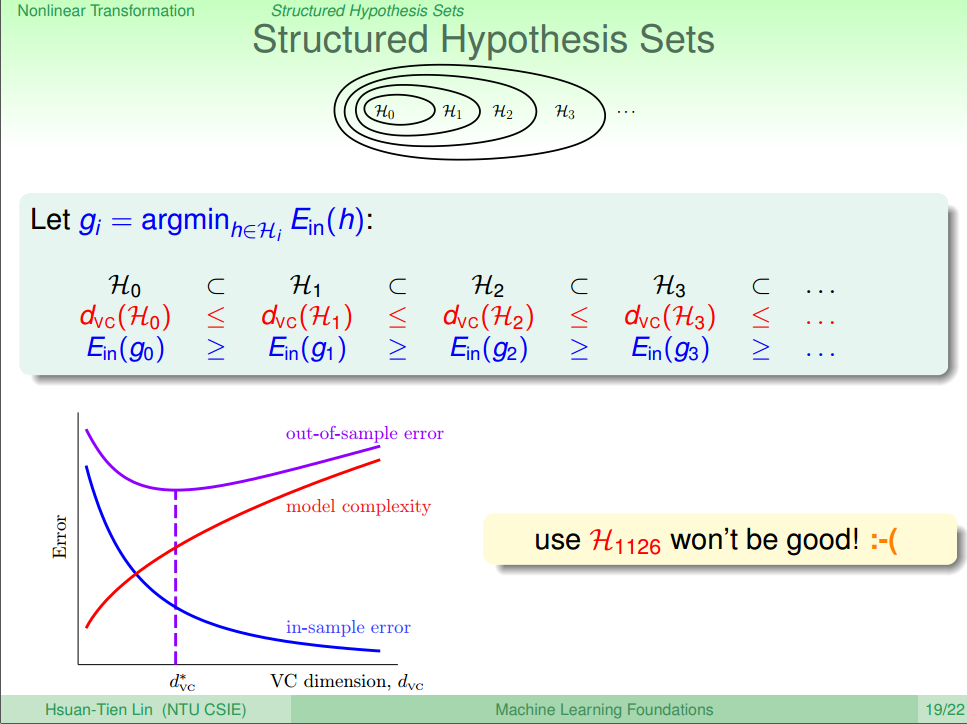# Summary

1. 首先介绍了非线性分类模型，通过非线性的特征变化，将非线性模型映射到一个线性空间进行相信分类。
2. 接着分析非线性模型的代价：时间和空间复杂度高，而且随着特征纬度的增加，模型的泛化能力变差
3. 最后我们通过数学分析得到如何能在使用非线性转化的过程中，尽可能的提高模型泛化能力：尽可能使用简单模型（低阶）。

# Reference

 机器学习基石(台湾大学-林轩田)\12\12 - 1 - Quadratic Hypothesis (23-47)

 机器学习基石(台湾大学-林轩田)\12\12 - 3 - Price of Nonlinear Transform (15-37)

 机器学习基石(台湾大学-林轩田)\12\12 - 4 - Structured Hypothesis Sets (09-36)

03-045131
10-13689
04-261万+
04-29180
04-1546
05-255421
03-24280
11-0570
01-1992
05-102万+
12-061739
11-13644
09-29829
09-30746
10-13745
10-13737
10-11716
10-13652
09-29647

### “相关推荐”对你有帮助么？

•非常没帮助
•没帮助
•一般
•有帮助
•非常有帮助被折叠的  条评论 为什么被折叠?到【灌水乐园】发言zhichengMLE

¥2 ¥4 ¥6 ¥10 ¥20余额支付 (余额：-- )扫码支付获取中扫码支付点击重新获取扫码支付1.余额是钱包充值的虚拟货币，按照1:1的比例进行支付金额的抵扣。
2.余额无法直接购买下载，可以购买VIP、C币套餐、付费专栏及课程。余额充值